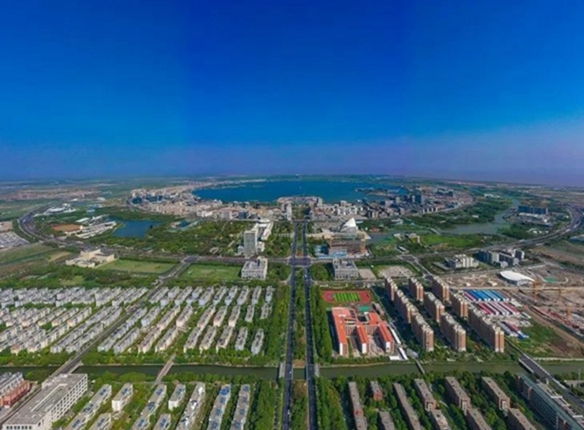## Lin-gang Special Area's 4 years by numbers: Overall

en.lingang.gov.cn| Updated: Aug 25, 2023A bird's-eye view of Dishui Lake, a landmark of the Lin-gang Special Area. [Photo by Xing Qianli]

21.2 percent

From 2019 to 2022, the average annual growth rate of gross regional domestic product was 21.2 percent.

38.9 percent

From 2019 to 2022, the average annual growth rate of the total output value of industries above a designated size was 37.8 percent. It viewed a 52 percent growth in the first half of this year, with two-year average growth of 38.9 percent.

404 billion yuan

From 2019 to 2022, the average annual growth of investment in fixed assets was 39.9 percent. In 2023, there was 57.2 percent growth in first half year. The two-year average growth was 39 percent. Over the past four years, total investment in fixed assets has totaled 404 billion yuan (\$55.4 billion).

40.5 percent

From 2019 to 2022, total merchandise sales above a designated limit grew at an average annual rate of 28.9 percent. There was a 55.2 percent increase in the first half of this year, with two-year average growth of 40.5 percent.

39.6 percent

The total value of imports and exports of the Yangshan Free Trade Zone has seen an average annual growth rate of 44 percent since its establishment in 2020. In the first half of this year, the growth rate was 54.6 percent, with an average growth rate of 39.6 percent over the last two years.

\$4.68 billion

From 2019 to 2022, the average annual growth rate of foreign investment was 103.7 percent. Since the establishment of the Lin-gang Special Area, the total foreign investment amounted to \$4.68 billion, of which \$863 million was realized from January to June this year.

29.4 percent

From 2019-2022, total tax revenue increased at an average annual rate of 16.2 percent. There was a 47.5 percent growth in the first half of this year, with two-year average growth of 29.4 percent.